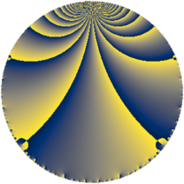Properties

 Label 99.3.lLevel $99$ Weight $3$ Character orbit 99.l Rep. character $\chi_{99}(26,\cdot)$ Character field $\Q(\zeta_{10})$ Dimension $32$ Newform subspaces $1$ Sturm bound $36$ Trace bound $0$

Related objects

Defining parameters

 Level: $$N$$ $$=$$ $$99 = 3^{2} \cdot 11$$ Weight: $$k$$ $$=$$ $$3$$ Character orbit: $$[\chi]$$ $$=$$ 99.l (of order $$10$$ and degree $$4$$) Character conductor: $$\operatorname{cond}(\chi)$$ $$=$$ $$33$$ Character field: $$\Q(\zeta_{10})$$ Newform subspaces: $$1$$ Sturm bound: $$36$$ Trace bound: $$0$$

Dimensions

The following table gives the dimensions of various subspaces of $$M_{3}(99, [\chi])$$.

Total New Old
Modular forms 112 32 80
Cusp forms 80 32 48
Eisenstein series 32 0 32

Trace form

 $$32 q + 16 q^{4} - 16 q^{7} + O(q^{10})$$ $$32 q + 16 q^{4} - 16 q^{7} + 48 q^{10} + 8 q^{13} + 96 q^{16} - 40 q^{19} - 60 q^{22} - 188 q^{25} - 348 q^{28} - 164 q^{31} + 296 q^{34} - 36 q^{37} + 48 q^{40} + 544 q^{43} + 296 q^{46} + 196 q^{49} - 640 q^{52} - 440 q^{55} - 208 q^{58} - 432 q^{61} - 328 q^{64} + 48 q^{67} + 112 q^{70} + 712 q^{73} + 2104 q^{76} + 432 q^{79} + 676 q^{82} - 68 q^{85} - 176 q^{88} + 64 q^{91} - 1360 q^{94} + 132 q^{97} + O(q^{100})$$

Decomposition of $$S_{3}^{\mathrm{new}}(99, [\chi])$$ into newform subspaces

Label Dim. $$A$$ Field CM Traces $q$-expansion
$a_{2}$ $a_{3}$ $a_{5}$ $a_{7}$
99.3.l.a $32$ $2.698$ None $$0$$ $$0$$ $$0$$ $$-16$$

Decomposition of $$S_{3}^{\mathrm{old}}(99, [\chi])$$ into lower level spaces

$$S_{3}^{\mathrm{old}}(99, [\chi]) \cong$$ $$S_{3}^{\mathrm{new}}(33, [\chi])$$$$^{\oplus 2}$$